# PSAT Math : How to find if right triangles are congruent

## Example Questions

### Example Question #521 : Geometry

You are given triangles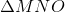and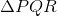,with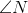and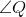both right angles, and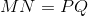. Which of these statements, along with what you are given, is not enough to prove that?

I)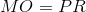II)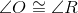III)andhave the same area.

Statement II only

None of the three statements is enough to prove congruence.

Any of the three statements is enough to prove congruence.

Statement III only

Statement I only

Any of the three statements is enough to prove congruence.

Explanation:, and the right angles areand, so we have two right triangles with congruent legs.

If we also know that, then the hypotenuses of the right triangles are also congruent, and this sets up the conditions of the Hypotenuse-Leg Theorem.

If we also know that, then, along with the fact that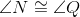(both being right angles) and nonincluded sides, the conditions of the Angle-Angle-Side Theorem are set up.

If we also knowandhave the same area, we can demonstrate that the other legs are congruent. The area of a right triangle is half the product of its legs, and since we have the same areas,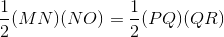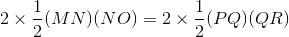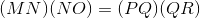Since,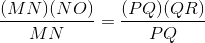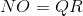The legs and the included angles (the right angles) are congruent, thus setting up the conditions for the Angle-Side-Angle Postulate.

In all three cases, congruence follows, so the correct response is "Any of the three statements is enough to prove congruence."